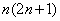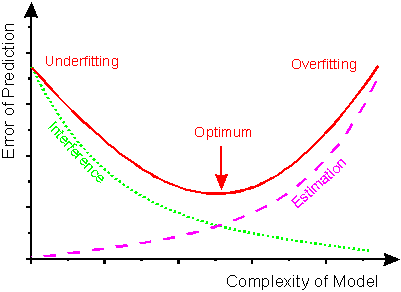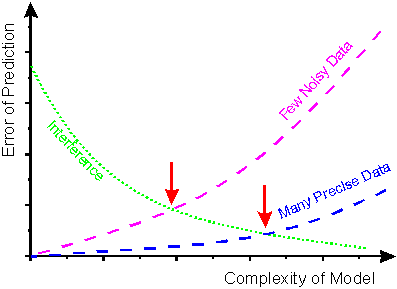Home News About Me Ph. D. Thesis Abstract Table of Contents 1. Introduction 2. Theory  Fundamentals of the Multivariate Data Analysis 2.1. Overview of the Multivariate Quantitative Data Analysis 2.2. Experimental Design 2.3. Data Preprocessing 2.4. Data Splitting and Validation 2.5. Calibration of Linear Relationships 2.6. Calibration of Nonlinear Relationships 2.7. Neural Networks  Universal Calibration Tools 2.8. Too Much Information Deteriorates Calibration 2.8.1. Overfitting, Underfitting and Model Complexity 2.8.2. Neural Networks and the Complexity Problem 2.8.3. Brute Force Variable Selection 2.8.4. Variable Selection by Stepwise Algorithms 2.8.5. Variable Selection by Genetic Algorithms 2.8.6. Variable Selection by Simulated Annealing 2.8.7. Variable Compression by Principal Component Analysis 2.8.8. Topology Optimization by Pruning Algorithms 2.8.9. Topology Optimization by Genetic Algorithms 2.8.10. Topology Optimization by Growing Neural Network Algorithms 2.9. Measures of Error and Validation 3. Theory  Quantification of the Refrigerants R22 and R134a: Part I 4. Experiments, Setups and Data Sets 5. Results  Kinetic Measurements 6. Results  Multivariate Calibrations 7. Results  Genetic Algorithm Framework 8. Results  Growing Neural Network Framework 9. Results  All Data Sets 10. Results  Various Aspects of the Frameworks and Measurements 11. Summary and Outlook 12. References 13. Acknowledgements Publications Research Tutorials Downloads and Links Contact Search Site Map Print this Page2.8.1.   Overfitting, Underfitting and Model Complexity

Neural networks are often referred to as universal function approximators since theoretically any continuous function can be approximated to a prescribed degree of accuracy by increasing the number of neurons in the hidden layer of a feedforward backpropagation network . This can be proven by Kolmogrov's theorem stating that a neural network with linear combinations ofmonotonically increasing nonlinear functions of only one variable is able to fit any continuous function of n variables . Yet in reality, the objective of a multivariate calibration is not to approximate a calibration data set with an ultimate accuracy, but to find a calibration with the best possible generalizing ability . The gap between the approximation of a calibration data set and the generalization ability of a calibration becomes the more problematic the higher the number of variables and the smaller the data set, which will be further explained in the following sections.

The best measure for the generalizing ability is the error of prediction of as many independent separate validation data as possible. According to figure 2 the error of prediction is composed of two main contributions, the remaining interference error and the estimation error . The interference error is the systematic error (bias) due to unmodeled interference in the data, as the calibration model is not complex enough to capture all the interferences of the relationship between sensor responses and analytes. The estimation error is caused by modeling measured random noise of various kinds. The optimal prediction is obtained, when the remaining interference error and the estimation error balance each other (arrow in figure 2). The effect of the prediction error increasing due to a too simple model is called underfitting whereas the effect of the increased prediction error due to a too complex model is called overfitting or overtraining. In figure 3 it is shown that the optimal complexity of the model highly depends on the size and quality of the calibration data set. For data sets, which are noisy and limited in size, a simple calibration model is needed to prevent the overfitting. Neural networks, which are too complex (too big), are in danger of learning these data by heart and consequently model noise of the data. For big data sets, which contain only little noise, the best model is more complex resulting in an overall smaller prediction error for the same functional relationship. Consequently, for each data set an optimal model complexity has to be found  whereby the complexity of the models is directly related with the number of variables utilized by the model. The search of the optimal models is a very difficult task in the field of the multivariate calibration and is further discussed in section 2.8.2.figure 2:    Scheme for the error of prediction as a function of the complexity of the calibration model.

An overfitting can be detected, if the error of prediction of the independent validation data is significantly higher than the error of prediction of the calibration data whereby both data sets have to be within the same range of the response variables (for example within the same concentration range) to prevent additional biases due to extrapolation . An underfitting manifests in high prediction errors for both data sets. Not only neural networks are affected by the effects of underfitting and overfitting, but also most modern multivariate calibration algorithms are subject to these effects . In the following section, the discussion of the construction of optimal model complexities mainly refers to neural networks but can also be generalized for various multivariate calibration methods in many topics.figure 3:    Scheme for the error of prediction depending on the size and quality of the calibration data set, which influence the estimation error.

 Page 48 © Frank Dieterle, 03.03.2019